# Challenges in exchange rate forecasting

Challenges in exchange rate forecasting

Challenges in forecasting of exchange rate:

Following are some of the challenges:

• Purchasing power parity, interest rate parity & international fisher effects can only show 10% of the changes or variations in the exchange rates.
• There is problem with underestimated situations & its influence on exchange rate variations.
• Small movements in exchange rate fluctuations can be predicted easily but large movements can’t be predicted with level of certainty.

Apart from this following are some of the exchange rate risks that make forecasting difficult:

• Transaction risk – this is a cash flow risk that deals with the effect of exchange rate moves – it influences to receivables, payables or repatriation of dividend.
• Translation risk – this is a balance sheet exchange risk – here the exchange rate moves to the valuation of foreign subsidiary’s balance sheet & in turn affects the balance sheet of the parent company.
• Economic risk – this is a risk to the present value of future operating cash flows from exchange rate movements. The economic risk has an influence on exchange rate on revenues i.e. domestic sales & exports & operating expenses i.e. cost of domestic inputs & imports.
Similar Homework Help Questions
• ### 2. If I use the market approach to exchange rate forecasting, which of the following would I use to predict future spot...

2. If I use the market approach to exchange rate forecasting, which of the following would I use to predict future spot rates. a. Past spot rates b. GNP differences between countries c. Forward Rates d. Today’s rate is the expected future rate e. Charting techniques 3. A large government deficit relative to GDP, a high rate of money expansion accompanied by fixed exchange rates, along with substantial government expenditures are some of the common characteristics of _________ risk. a.exchange...

• ### Question 2 (a) Explain the difference between nominal exchange rate and real exchange rate (6) As mentioned in clas...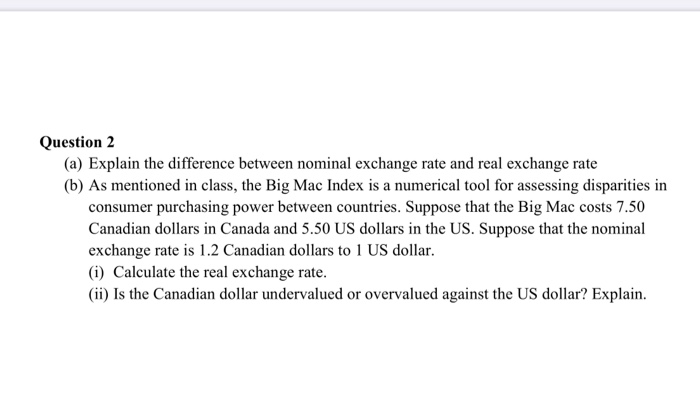Question 2 (a) Explain the difference between nominal exchange rate and real exchange rate (6) As mentioned in class, the Big Mac Index is a numerical tool for assessing disparities in consumer purchasing power between countries. Suppose that the Big Mac costs 7.50 Canadian dollars in Canada and 5.50 US dollars in the US. Suppose that the nominal exchange rate is 1.2 Canadian dollars to 1 US dollar. (i) Calculate the real exchange rate. (ii) Is the Canadian dollar undervalued...

• ### What happens to the fundamental value of a country's exchange money supply in a fixed-exchange-rate system? Does th...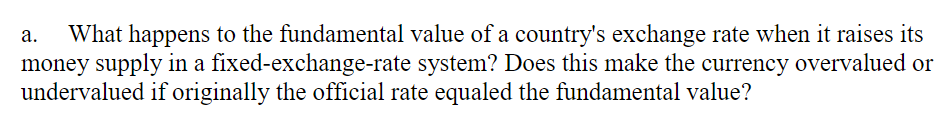What happens to the fundamental value of a country's exchange money supply in a fixed-exchange-rate system? Does this make the currency overvalued or undervalued if originally the official rate equaled the fundamental value? rate when it raises its а. What happens to the fundamental value of a country's exchange money supply in a fixed-exchange-rate system? Does this make the currency overvalued or undervalued if originally the official rate equaled the fundamental value? rate when it raises its а.

• ### Suppose that the current spot exchange rate is 0.80/\$ and the three-month forward exchange rate i...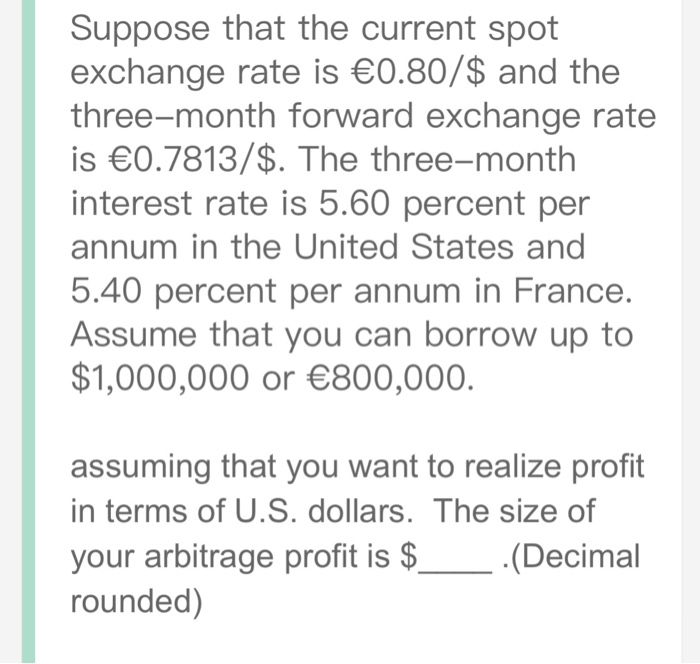Suppose that the current spot exchange rate is 0.80/\$ and the three-month forward exchange rate is 0.7813/\$. The three-month interest rate is 5.60 percent per annum in the United States and 5.40 percent per annum in France. Assume that you can borrow up to \$1,000,000 or 800,000. assuming that you want to realize profit in terms of U.S. dollars. The size of your arbitrage profit is S rounded) Suppose that the current spot exchange rate is 0.80/\$ and the three-month...

• ### Suppose that the current spot exchange rate is €0.8250/\$ and the three month forward exchange rate...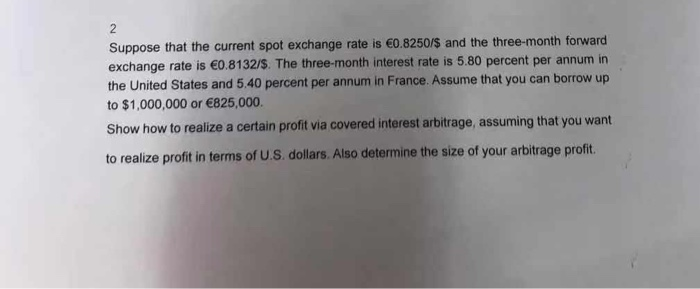Suppose that the current spot exchange rate is €0.8250/\$ and the three month forward exchange rate is €0.8132/\$. The three-month interest rate is 5.80 percent per annum in the United States and 5.40 percent per annum in France. Assume that you can borrow up to \$1,000,000 or €825,000. Show how to realize a certain profit via covered interest arbitrage, assuming that you want to realize profit in terms of U.S. dollars. Also determine the size of your arbitrage profit

• ### 6. The current exchange rate is \$1.00 = 1.50 Swiss franc. The exchange rate is expected to be \$1.00 = 1.25 Swiss fr...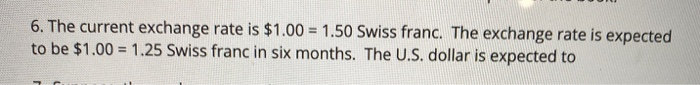6. The current exchange rate is \$1.00 = 1.50 Swiss franc. The exchange rate is expected to be \$1.00 = 1.25 Swiss franc in six months. The U.S. dollar is expected to

• ### Nominal Country Forward Inflation rate Exchange rate differential interest rate CHF 1.5% нKD 3.5% Differential: Based...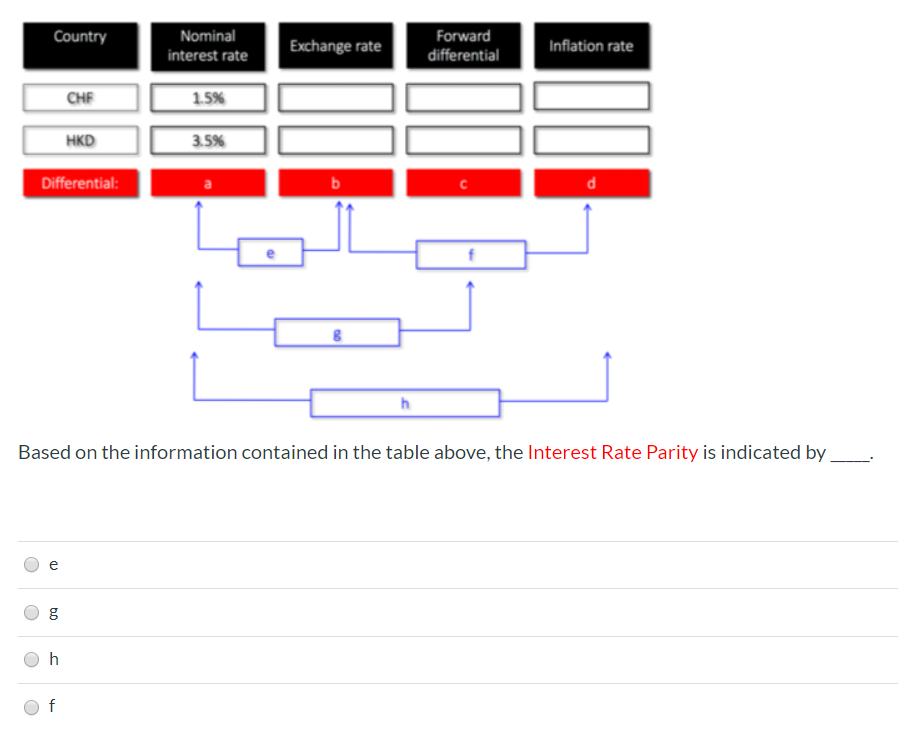Nominal Country Forward Inflation rate Exchange rate differential interest rate CHF 1.5% нKD 3.5% Differential: Based on the information contained in the table above, the Interest Rate Parity is indicated by е f

• ### Introduction to Forecasting:a. What is forecasting a. Usefulness & relevance of business forecasting b. Types of for...

Introduction to Forecasting:a. What is forecasting a. Usefulness & relevance of business forecasting b. Types of forecasting c. Areas of application of forecasting d. Limitations of business forecasting e. Business forecasting software f. Business Time Series Data

• ### Foundations of Financial Management: Define and discuss what an exchange rate is and how exchange rates impact international business where firms do business in several currencies worldwide

Foundations of Financial Management: Define and discuss what an exchange rate is and how exchange rates impact international business where firms do business in several currencies worldwide

• ### If the current exchange rate is higher than the Fed's target exchange rate, the Fed would O A. implement interest r...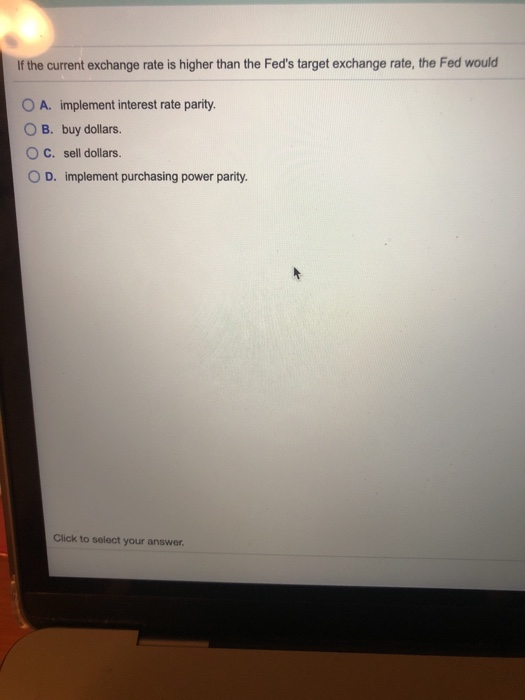If the current exchange rate is higher than the Fed's target exchange rate, the Fed would O A. implement interest rate parity. O B. buy dollars. O C. sell dollars. OD. implement purchasing power parity. Click to select your answer.

Need Online Homework Help?